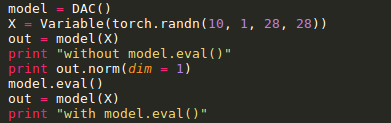# LRN not working properly

Hi
I have one query regarding the functioning of the LRN layer. I use the LRN layer to normalize my tensors to unit norm. During training it generates unit norm tensors.However during test phase, it doesn’t generate unit norm vectors as i use model.eval(). Can somebody please explain what is the reason behind this? and how can this be avoided?This is the code snippet
""
self.lrn = nn.LocalResponseNorm(size=2 *10 + 1, alpha=2 * 10 + 1, beta=0.5, k=1e-4)

model = DAC()
X = Variable(torch.randn(3, 1, 28, 28))
out = model(X)
print "without model.eval()"
print out.norm(dim = 1)
model.eval()
out = model(X)
print "with model.eval()“
print out.norm(dim = 1)